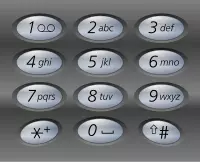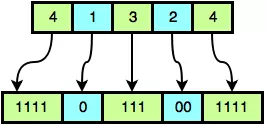# Lombok 笔记

## Lombok使用

Lombok 可以通过注解的形式，来帮助我们在开发的时候省略掉一些重复的工作。比如设置 get、set 方法，设置无参构造函数或者全参构造函数。

# Median of Two Sorted Arrays 双数组中位数

## 题目

### 4. Median of Two Sorted Arrays

There are two sorted arrays nums1 and nums2 of size m and n respectively. Find the median of the two sorted arrays. The overall run time complexity should be O(log (m+n)).

# Letter Combinations of a Phone Number 手机键盘输入组合

## 题目

### 17. Letter Combinations of a Phone Number

Given a digit string, return all possible letter combinations that the number could represent. A mapping of digit to letters (just like on the telephone buttons) is given below.# What's Next?

## 题目

### What’s Next?

Johnny is playing with a large binary number, B. The number is so large that it needs to be compressed into an array of integers, A, where the values in even indices `(0, 2, 4,...)` represent some number of consecutive `1` bits and the values in odd indices `(1, 3, 5,...)` represent some number of consecutive `0` bits in alternating substrings of B.

For example, suppose we have array A = `{4, 1, 3, 2, 4}`. A0 represents `"1111"`, A1 represents `"0"`, A2 represents `"111"`, A3 represents `"00"`, and A4 represents `"1111"`. The number of consecutive binary characters in ith the substring of B corresponds to integer Ai, as shown in this diagram:When we assemble the sequential alternating sequences of `1`‘s and `0`‘s, we get B = `11110111001111`.

We define setCount(B) to be the number of `1`‘s in a binary number, B. Johnny wants to find a binary number, D, that is the smallest binary number > B where setCount(B) = setCount(D). He then wants to compress D into an array of integers, C (in the same way that integer array A contains the compressed form of binary string B).

Johnny isn’t sure how to solve the problem. Given array A, find integer array C and print its length on a new line. Then print the elements of array C as a single line of space-separated integers.